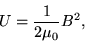# What is the RMS magnetic field of light

• lloyd21

## Homework Statement

A window, 1.0m by 1.0m receives 27J of energy, from sunlight, in one minute. What is the Rms magnetic field of the light striking the window.

## Homework Equations

E = cB
I = (ErmsBrms) / μo

## The Attempt at a Solution

Not sure if those equations are right when solving this problem and can't find a problem similar to it...completely stuck. Do I use Poynting's vector?

For a homogeneous magnetic field within some volume, what is its energy density?
For an electromagnetic field satisfying E=cB, what is the associated energy density of the electric field?

How can you convert the given area and time to a volume, and why does the approach with a homogeneous field work?

Energy Density = B^2 / Mew ??

µ (mu)?

##\displaystyle \frac {B^2} {2 \mu}## is the energy density of magnetic fields.

Yes I get that, I don't know how I would find the magnetic field. The area is 1. Not to sure how that and time can get a volume either.

I first found the intensity I = (27 J) / (60s)(1) = 0.45 W/m2

If I= EoBo / 2Mu

then Bo = 2Mu I / Eo

So Bo = 2(4pie x 10^-7 Tm/A) (0.45 W/m^2) / (8.854x10^-12 F/m)

Bo = 1.2x 10^5

Brms = 1.2 x 10^5 / sqrt(2) = 9.0 x 10^4

Other than my final units which I don't know yet, does that look correct??

If I= EoBo / 2Mu
Where does that equation come from? What are "Eo" and "Bo"?

Eo is Electric Field of 8.854x10^-12
Bo is the magnetic field I would solve for? then turn into Brms by dividing my answer by sqrt(2) ...?

Eo is Electric Field of 8.854x10^-12
That doesn't make sense.
The electric field strength does not have a fixed value in any unit system.
The electric vacuum permittivity has this numerical value in a suitable unit system, if you add those units. It is not the electric field strength.

Stop multiplying random numbers please, this does not help.
I gave hints how to approach the problem in post 2, you can follow them if you like. So far you just followed the first hint given there.

Yes, number 1.2. I don't know the magnetic field B, c is the speed of light in a vacuum constant?
3. I don't know how the area and time can give volume.

2. I don't know the magnetic field B, c is the speed of light in a vacuum constant?
B is B. Yes c is the speed of light in vacuum (and to a good approximation the speed of light in air).
3. I don't know how the area and time can give volume.
You know the speed.

https://s.yimg.com/hd/answers/i/a22e6906e4a44a42b9eac01e38e8e649_A.png?a=answers&mr=0&x=1449097211&s=669a9de4864363846733969a48433616 [Broken] This is what I initially used...are these correct or incorrect

Last edited by a moderator:
You can use the Poynting vector, sure, but you have to find some way to eliminate unknown quantities (like the electric field) out of that equation.
The relation between electric and magnetic field is in post 1 already.

Im more confused now than when I started...I have no idea

wait, the volume times the energy should give me a total energy density. Then multiply that by Mew, and sqrt it...that will give my rms magnetic field!?